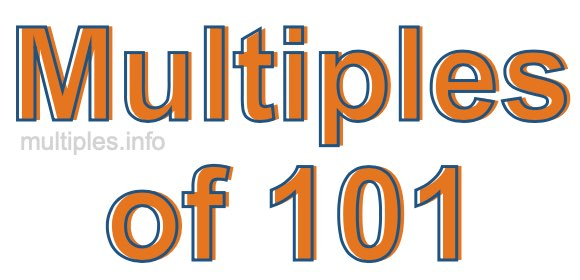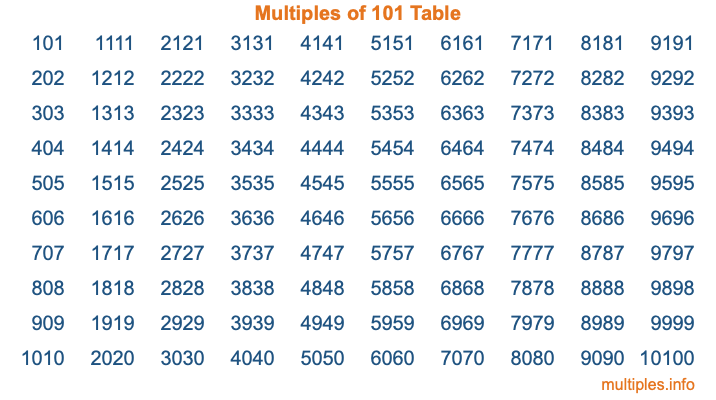Multiples of 101Welcome to the Multiples of 101 page. Here we will first teach you everything you will ever need to know about the multiples of 101, and then give you a study guide summary of everything we taught you to make sure you remember it all. Use this page to look up facts and learn information about the multiples of 101. This page will make you a multiples of one hundred one expert!

Definition of Multiples of 101
Multiples of 101 are all the numbers that when divided by 101 equal an integer. Each of the multiples of 101 are called a multiple. A multiple of 101 is created by multiplying 101 by an integer.

Therefore, to create a list of multiples of 101, you start with 1 multiplied by 101, then 2 multiplied by 101, then 3 multiplied by 101, and so on for as long as you want. Thus, the list of the first five multiples of 101 is 101, 202, 303, 404, and 505. To see a larger list of multiples of 101, see the printable image of Multiples of 101 further down on this page. We also have a category where you can choose any nth multiple of 101.

Multiples of 101 Checker
The Multiples of 101 Checker below checks to see if any number of your choice is a multiple of 101. In other words, it checks to see if there is any number (integer) that when multiplied by 101 will equal your number. To do that, we divide your number by 101. If the the quotient is an integer, then your number is a multiple of 101.

Is  a multiple of 101?

Least Common Multiple of 101 and ...
A Least Common Multiple (LCM) is the lowest multiple that two or more numbers have in common. This is also called the smallest common multiple or lowest common multiple and is useful to know when you are adding our subtracting fractions. Enter one or more numbers below (101 is already entered) to find the LCM.

Check out our LCM Calculator if you need more details about the Least Common Multiple or if you need the LCM for different numbers for adding and subtraction fractions.

nth Multiple of 101
As we stated above, 101 is the first multiple of 101, 202 is the second multiple of 101, 303 is the third multiple of 101, and so on. Enter a number below to find the nth multiple of 101.

th multiple of 101

Multiples of 101 vs Factors of 101
101 is a multiple of 101 and a factor of 101, but that is where the similarities end. All postive multiples of 101 are 101 or greater than 101. All positive factors of 101 are 101 or less than 101.

Below is the beginning list of multiples of 101 and the factors of 101 so you can compare:

Multiples of 101: 101, 202, 303, 404, 505, etc.

Factors of 101: 1, 101

As you can see, the multiples of 101 are all the numbers that you can divide by 101 to get a whole number. The factors of 101, on the other hand, are all the whole numbers that you can multiply by another whole number to get 101.

It's also interesting to note that if a number (x) is a factor of 101, then 101 will also be a multiple of that number (x).

Multiples of 101 vs Divisors of 101
The divisors of 101 are all the integers that 101 can be divided by evenly. Below is a list of the divisors of 101.

Divisors of 101: 1, 101

The interesting thing to note here is that if you take any multiple of 101 and divide it by a divisor of 101, you will see that the quotient is an integer.

Multiples of 101 Table
Below is an image of the first 100 multiples of 101 in a table. The table is in chronological order, column by column. The first column has the first ten multiples of 101, the second column has the next ten multiples of 101, and so on.The Multiples of 101 Table is also referred to as the 101 Times Table or Times Table of 101. You are welcome to print out our table for your studies.

Negative Multiples of 101
Although not often discussed or needed in math, it is worth mentioning that you can make a list of negative multiples of 101 by multiplying 101 by -1, then by -2, then by -3, and so on, to get the following list of negative multiples of 101:

-101, -202, -303, -404, -505, etc.

Multiples of 101 Summary
Below is a summary of important Multiples of 101 facts that we have discussed on this page. To retain the knowledge on this page, we recommend that you read through the summary and explain to yourself or a study partner why they hold true.

There are an infinite number of multiples of 101.

A multiple of 101 divided by 101 will equal a whole number.

101 divided by a factor of 101 equals a divisor of 101.

The nth multiple of 101 is n times 101.

The largest factor of 101 is equal to the first positive multiple of 101.

101 is a multiple of every factor of 101.

101 is a multiple of 101.

A multiple of 101 divided by a divisor of 101 equals an integer.

101 divided by a divisor of 101 equals a factor of 101.

Any integer times 101 will equal a multiple of 101.

Multiples of a Number
Here you can get the multiples of another number, all with the same attention to detail as we did for multiples of 101 on this page.

Multiples of
Multiples of 102
Did you find our page about multiples of one hundred one educational? Do you want more knowledge? Check out the multiples of the next number on our list!